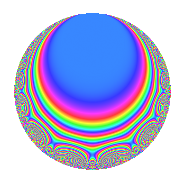# Properties

 Label 85.2.cLevel 85 Weight 2 Character orbit c Rep. character $$\chi_{85}(84,\cdot)$$ Character field $$\Q$$ Dimension 8 Newform subspaces 1 Sturm bound 18 Trace bound 0

# Related objects

## Defining parameters

 Level: $$N$$ = $$85 = 5 \cdot 17$$ Weight: $$k$$ = $$2$$ Character orbit: $$[\chi]$$ = 85.c (of order $$2$$ and degree $$1$$) Character conductor: $$\operatorname{cond}(\chi)$$ = $$85$$ Character field: $$\Q$$ Newform subspaces: $$1$$ Sturm bound: $$18$$ Trace bound: $$0$$

## Dimensions

The following table gives the dimensions of various subspaces of $$M_{2}(85, [\chi])$$.

Total New Old
Modular forms 12 12 0
Cusp forms 8 8 0
Eisenstein series 4 4 0

## Trace form

 $$8q - 16q^{4} + O(q^{10})$$ $$8q - 16q^{4} - 16q^{15} + 24q^{16} + 16q^{19} + 8q^{21} - 24q^{26} + 32q^{30} + 32q^{34} - 24q^{35} - 56q^{36} - 16q^{49} - 24q^{50} - 40q^{51} + 8q^{55} + 48q^{59} + 88q^{60} - 48q^{64} + 88q^{66} + 8q^{69} - 8q^{70} - 32q^{76} - 16q^{81} - 72q^{84} + 8q^{85} + 24q^{86} - 24q^{89} - 104q^{94} + O(q^{100})$$

## Decomposition of $$S_{2}^{\mathrm{new}}(85, [\chi])$$ into newform subspaces

Label Dim. $$A$$ Field CM Traces $q$-expansion
$$a_2$$ $$a_3$$ $$a_5$$ $$a_7$$
85.2.c.a $$8$$ $$0.679$$ 8.0.$$\cdots$$.11 None $$0$$ $$0$$ $$0$$ $$0$$ $$q-\beta _{1}q^{2}-\beta _{4}q^{3}+(-2+\beta _{3})q^{4}+(-\beta _{2}+\cdots)q^{5}+\cdots$$